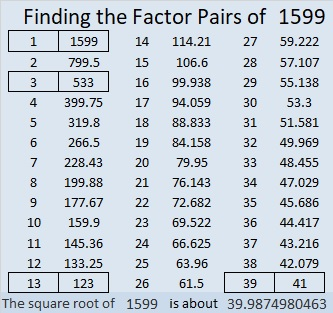# 1599 and Level 5

Contents

### Today’s Puzzle:

Write the numbers 1 to 10 in both the first column and the top row so that this level 5 puzzle will function like a multiplication table. Use logic with every step.### Factors of 1599:

• 1599 is a composite number.
• Prime factorization: 1599 = 3 × 13 × 41.
• 1599 has no exponents greater than 1 in its prime factorization, so √1599 cannot be simplified.
• The exponents in the prime factorization are 1, 1, and 1. Adding one to each exponent and multiplying we get (1 + 1)(1 + 1)(1 + 1) = 2 × 2 × 2 = 8. Therefore 1599 has exactly 8 factors.
• The factors of 1599 are outlined with their factor pair partners in the graphic below.### More about the Number 1599:

1599 is the hypotenuse of FOUR Pythagorean triples:
276-1575-1599, which is 3 times (92-525-533),
351-1560-1599, which is 39 times (9-40-41),
615-1476-1599, which is (5-12-13) times 123, and
924-1305-1599, which is 3 times (308-435-533).

1599 is the difference of two squares four different ways:
800² – 799² = 1599,
268² – 265² = 1599,
68² – 55² = 1599, and
40² – 1² = 1599.
Yes, we are just one number away from a perfect square!

This site uses Akismet to reduce spam. Learn how your comment data is processed.